Next: Gravity Waves in Deep Up: Waves in Incompressible Fluids Previous: Introduction

# Gravity Waves

Consider a stationary body of water, of uniform depth, located on the surface of the Earth. This body is assumed to be sufficiently small compared to the Earth that its unperturbed surface is approximately planar. Let the Cartesian coordinatemeasure vertical height, withcorresponding to the aforementioned surface. Suppose that a small amplitude wave propagates horizontally through the water, and letbe the associated velocity field.

Because water is essentially incompressible, its equations of motion are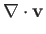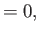(11.1)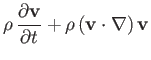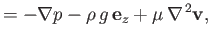(11.2)

whereis the (uniform) mass density,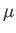the (uniform) viscosity, and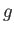the (uniform) acceleration due to gravity. (See Section 1.14.) Let us write(11.3)

where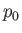is atmospheric pressure, and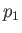the pressure perturbation due to the wave. Of course, in the absence of the wave, the water pressure a depthbelow the surface is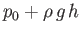. (See Chapter 2.) Substitution into Equation (11.2) yields(11.4)

where we have neglected terms that are second order in small quantities (i.e., terms of order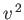).

Let us also neglect viscosity, which is a good approximation provided that the wavelength is not ridiculously small. [For instance, for gravity waves in water, viscosity is negligible as long as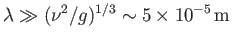.] It follows that(11.5)

Taking the curl of this equation, we obtain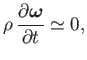(11.6)

where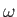is the vorticity. We conclude that the velocity field associated with the wave is irrotational. Consequently, the previous equation is automatically satisfied by writing(11.7)

where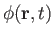is the velocity potential. (See Section 4.15.) However, from Equation (11.1), the velocity field is also divergence free. It follows that the velocity potential satisfies Laplace's equation,(11.8)

Finally, Equations (11.5) and (11.7) yield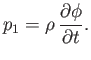(11.9)

We now need to derive the physical constraints that must be satisfied at the water's upper and lower boundaries. It is assumed that the water is bounded from below by a solid surface located at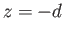. Because the water must always remain in contact with this surface, the appropriate physical constraint at the lower boundary is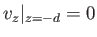(i.e., the normal velocity is zero at the lower boundary), or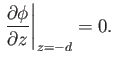(11.10)

The water's upper boundary is a little more complicated, because it is a free surface. Letrepresent the vertical displacement of this surface due to the wave. It follows that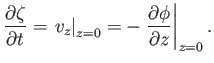(11.11)

The appropriate physical constraint at the upper boundary is that the water pressure there must equal atmospheric pressure, because there cannot be a pressure discontinuity across a free surface (in the absence of surface tension--see Section 11.11). Accordingly, from Equation (11.3), we obtain(11.12)

or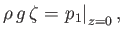(11.13)

which implies that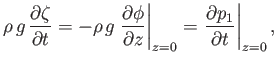(11.14)

where use has been made of Equation (11.11). The previous expression can be combined with Equation (11.9) to give the boundary condition(11.15)

Let us search for a wave-like solution of Equation (11.8) of the form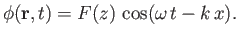(11.16)

This solution actually corresponds to a propagating plane wave of wave vector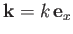, angular frequency, and amplitude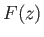(Fitzpatrick 2013). Substitution into Equation (11.8) yields(11.17)

whose independent solutions are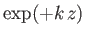and. Hence, a general solution to Equation (11.8) takes the form(11.18)

whereand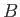are arbitrary constants. The boundary condition (11.10) is satisfied provided that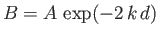, giving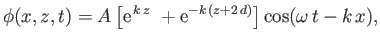(11.19)

The boundary condition (11.15) then yields(11.20)

which reduces to the dispersion relation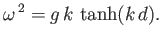(11.21)

The type of wave described in this section is known as a gravity wave.Next: Gravity Waves in Deep Up: Waves in Incompressible Fluids Previous: Introduction
Richard Fitzpatrick 2016-03-31